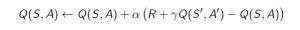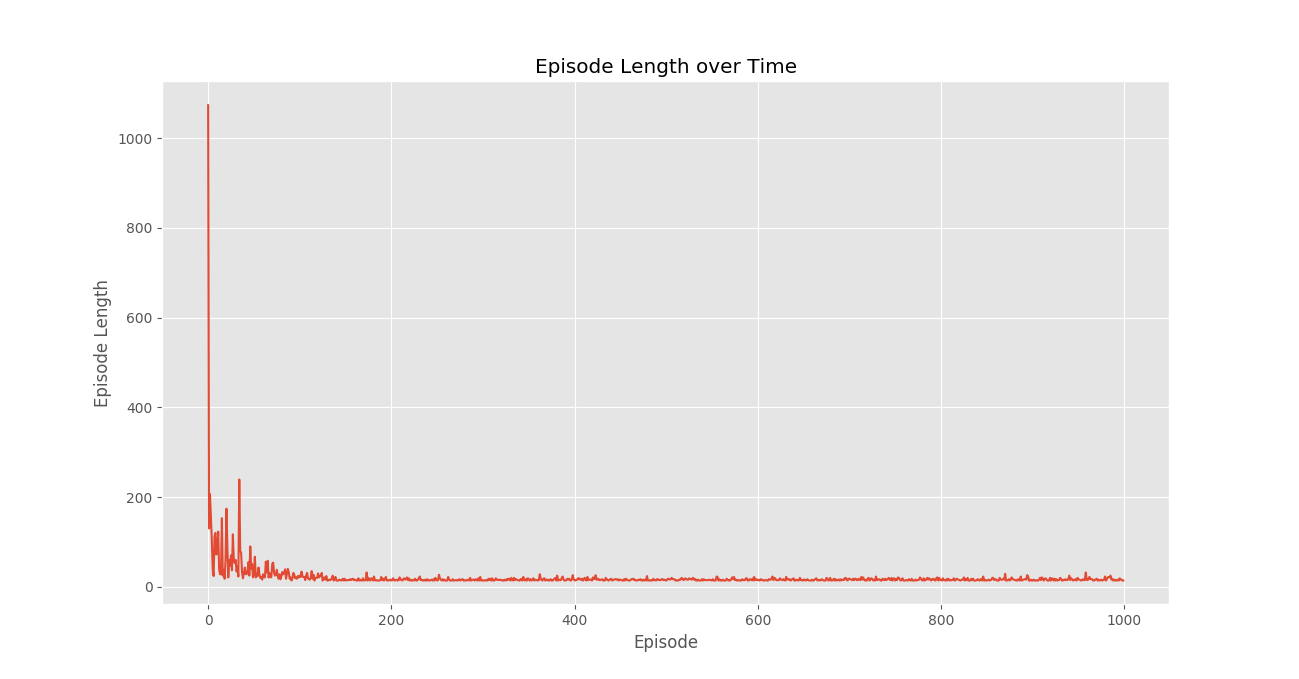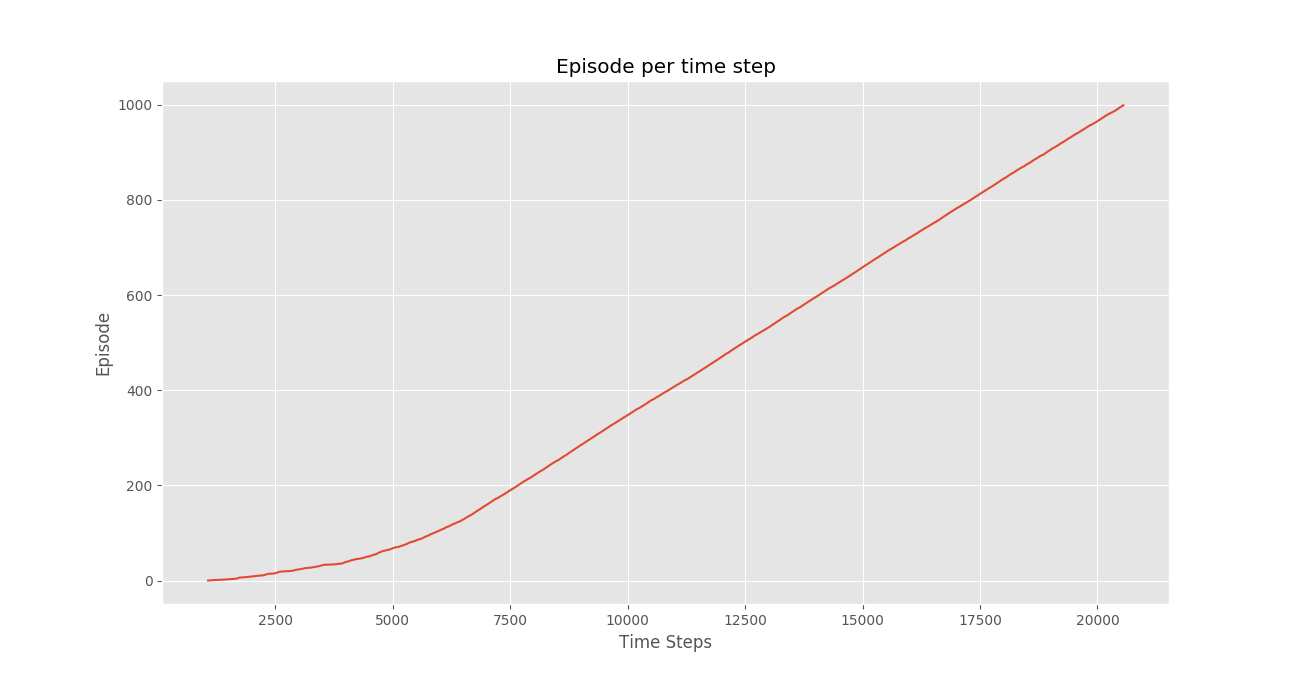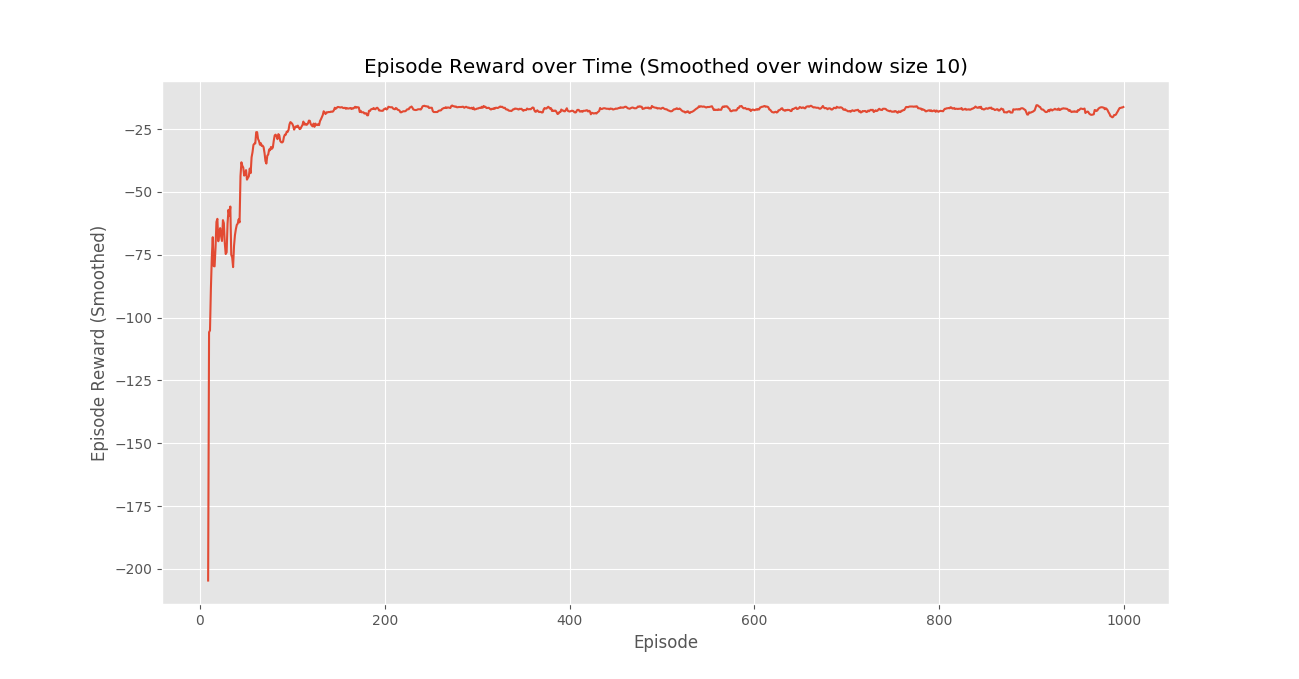# Q-Learning in Python

Pre-Requisite : Reinforcement Learning

Reinforcement Learning briefly is a paradigm of Learning Process in which a learning agent learns, overtime, to behave optimally in a certain environment by interacting continuously in the environment. The agent during its course of learning experience various different situations in the environment it is in. These are called states. The agent while being in that state may choose from a set of allowable actions which may fetch different rewards(or penalties). The learning agent overtime learns to maximize these rewards so as to behave optimally at any given state it is in.

Q-Learning is a basic form of Reinforcement Learning which uses Q-values (also called action values) to iteratively improve the behavior of the learning agent.

1. Q-Values or Action-Values: Q-values are defined for states and actions.is an estimation of how good is it to take the actionat the state. This estimation ofwill be iteratively computed using the TD- Update rule which we will see in the upcoming sections.
2. Rewards and Episodes: An agent over the course of its lifetime starts from a start state, makes a number of transitions from its current state to a next state based on its choice of action and also the environment the agent is interacting in. At every step of transition, the agent from a state takes an action, observes a reward from the environment, and then transits to another state. If at any point of time the agent ends up in one of the terminating states that means there are no further transition possible. This is said to be the completion of an episode.
3. Temporal Difference or TD-Update:

The Temporal Difference or TD-Update rule can be represented as follows :This update rule to estimate the value of Q is applied at every time step of the agents interaction with the environment. The terms used are explained below. :

•: Current State of the agent.
•: Current Action Picked according to some policy.
•: Next State where the agent ends up.
•: Next best action to be picked using current Q-value estimation, i.e. pick the action with the maximum Q-value in the next state.
•: Current Reward observed from the environment in Response of current action.
•(>0 and <=1) : Discounting Factor for Future Rewards. Future rewars are less valuable than current rewards so they must be discounted. Since Q-value is an estimation of expected rewards from a state, discounting rule applies here as well.
•: Step length taken to update the estimation of Q(S, A).
4. Choosing the Action to take using-greedy policy:-greedy policy of is a very simple policy of choosing actions using the current Q-value estimations. It goes as follows :

• With probabilitychoose the action which has the highest Q-value.
• With probabilitychoose any action at random.
5.

Now with all the theory required in hand let us take an example. We will use OpenAI’s gym environment to train our Q-Learning model.

Command to Install gym

pip install gym

Before starting with example, you will need some helper code in order to visualize the working of the algorithms. There will be two helper files which need to be downloaded in the working directory. One can find the files here.

Step # 1 : Import required libraries.

 import gym  import itertools  import matplotlib  import matplotlib.style  import numpy as np  import pandas as pd  import sys        from collections import defaultdict  from windy_gridworld import WindyGridworldEnv  import plotting     matplotlib.style.use('ggplot')

Step #2 : Create gym environment.

 env = WindyGridworldEnv()

Step #3 : Make the-greedy policy.

 def createEpsilonGreedyPolicy(Q, epsilon, num_actions):      """      Creates an epsilon-greedy policy based      on a given Q-function and epsilon.              Returns a function that takes the state      as an input and returns the probabilities      for each action in the form of a numpy array       of length of the action space(set of possible actions).      """     def policyFunction(state):              Action_probabilities = np.ones(num_actions,                  dtype = float) * epsilon / num_actions                             best_action = np.argmax(Q[state])          Action_probabilities[best_action] += (1.0 - epsilon)          return Action_probabilities          return policyFunction

Step #4 : Build Q-Learning Model.

 def qLearning(env, num_episodes, discount_factor = 1.0,                              alpha = 0.6, epsilon = 0.1):      """      Q-Learning algorithm: Off-policy TD control.      Finds the optimal greedy policy while improving      following an epsilon-greedy policy"""             # Action value function      # A nested dictionary that maps      # state -> (action -> action-value).      Q = defaultdict(lambda: np.zeros(env.action_space.n))          # Keeps track of useful statistics      stats = plotting.EpisodeStats(          episode_lengths = np.zeros(num_episodes),          episode_rewards = np.zeros(num_episodes))                  # Create an epsilon greedy policy function      # appropriately for environment action space      policy = createEpsilonGreedyPolicy(Q, epsilon, env.action_space.n)              # For every episode      for ith_episode in range(num_episodes):                      # Reset the environment and pick the first action          state = env.reset()                      for t in itertools.count():                              # get probabilities of all actions from current state              action_probabilities = policy(state)                  # choose action according to               # the probability distribution              action = np.random.choice(np.arange(                        len(action_probabilities)),                         p = action_probabilities)                  # take action and get reward, transit to next state              next_state, reward, done, _ = env.step(action)                  # Update statistics              stats.episode_rewards[ith_episode] += reward              stats.episode_lengths[ith_episode] = t                              # TD Update              best_next_action = np.argmax(Q[next_state])                  td_target = reward + discount_factor * Q[next_state][best_next_action]              td_delta = td_target - Q[state][action]              Q[state][action] += alpha * td_delta                  # done is True if episode terminated                 if done:                  break                                 state = next_state              return Q, stats

Step #5 : Train the model.

 Q, stats = qLearning(env, 1000)

Step #6 : Plot important statistics.

 plotting.plot_episode_stats(stats)Conclusion:
We see that in the Episode Reward over time plot that the episode rewards progressively increase over time and ultimately levels out at a high reward per episode value which indicates that the agent has learnt to maximize its total reward earned in an episode by behaving optimally at every state.

Attention geek! Strengthen your foundations with the Python Programming Foundation Course and learn the basics.

To begin with, your interview preparations Enhance your Data Structures concepts with the Python DS Course.

My Personal Notes arrow_drop_upIf you like GeeksforGeeks and would like to contribute, you can also write an article using contribute.geeksforgeeks.org or mail your article to contribute@geeksforgeeks.org. See your article appearing on the GeeksforGeeks main page and help other Geeks.

Please Improve this article if you find anything incorrect by clicking on the "Improve Article" button below.

Improved By : VishvajeetRamanuj